Refer to our Texas Go Math Grade 5 Answer Key Pdf to score good marks in the exams. Test yourself by practicing the problems from Texas Go Math Grade 5 Lesson 1.1 Answer Key Properties.

Unlock the Problem

The table shows the number of bones in several parts of the human body. What is the total number of bones in the ribs, the skull, and the spine?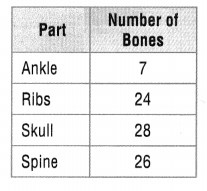To find the sum of addends using mental math, you can use the Commutative and Associative Properties.

Use properties to find 24 + 28 + 26.
24 + 28 + 26 = 28 + ________ + 26 Use the ______________ Property to reorder the addends.
= 28 + (24 + ________) Use the _____________ Property to group the addends.
= 28 + ________ Use mental math to add.
= ________
So, there are ________ bones in the ribs, the skull, and the spine.
It is given that
The table shows the number of bones in several parts of the human body
Now,
The given table is:Now,
From the given table,
We can observe that
The total number of bones in the ribs, the skull, and the spine = 24 + 28 + 26
Now,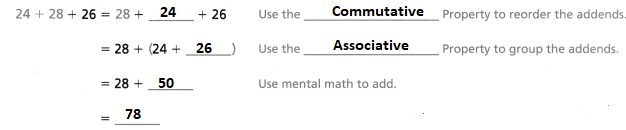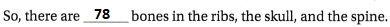Hence, from the above,
We can conclude that
The total number of bones in the ribs, the skull and the spine are: 78 bones

Math Talk
Mathematical Processes

Explain why grouping 24 and 26 makes the problem easier to solve.
The given table is:Now,
From the given table,
We can observe that
The number of bones in the ribs is: 24 bones
The number of bones in the skull is: 28 bones
The number of bones in the Spine is: 26 bones
Now,
If we add 24 and 28, then we will get 52
If we add 28 and 26, then we will get 54
If we add 24 and 26, then we will get 50
Now,
When we observe the addition values of 24 and 28, and 28 and 26, it will be difficult to do mental calculation
But,
When we observe the addition value of 24 and 26, the result is 50 which is the multiple of 10. So, it will be easy to do mental calculation
Hence, from the above,
We can conclude that
The grouping of 24 and 26 makes the problem easier because the result of grouping will be the multiple of 10

Example

Use the Distributive Property to find the product.
One Way
8 × 59 = 8 × (50 + 9) Use a multiple of 10 to write 59 as a sum.
= (8 × 50) + (8 × 9)    Use the Distributive Property.
= 400 + 72                 Use mental math to multiply.
= 472                         Use mental math to add.

Another Way
Use subtraction.
8 × 59 = 8 × (60 – 1) Use a multiple of 10 to write 59 as a difference.
= (8 × 60) – (8 × 1)    Use the Distributive Property.
= 480 – 8                   Use mental math to multiply.
= 472                         Use mental math to subtract.

Math Talk
Mathematical Processes

Explain how you could find the product 3 × 299 by using mental math.
The given multiplication expression is: 3 × 299
Now,
One way:
3 × 299 = 3 × (300 – 1)
= (3 × 300) – (3 × 1)
= 900 – 3
= 897
Other way:
3 × 299 = 3 × (200 + 99)
= (3 × 200) + (3 × 99)
= 600 + 297
= 897
Hence, from the above,
We can conclude that
The value for the given multiplication expression is: 897

Share and Show

Question 1.
Use properties to find 4 × 23 × 25.
23 × ______ × 25 __________ Property of Multiplication
23 × (_______ × ________) __________ Property of Multiplication
23 × _______
________
The given multiplication expression is: 4 × 23 × 25
Now,
The given multiplication can also be written as: 23 × 4 × 25
Now,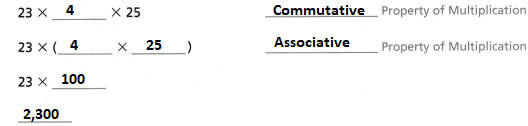Use properties to find the sum or product.

Question 2.
89 + 27 + 11
The given addition expression is: 89 + 27 + 11
Now,
89 + 27 + 11
= 27 + 89 + 11                         Commutative Property of addition
= 27 + (89 + 11)                       Associative Property of Addition
= 27 + 100                                Mental Math
= 127
Hence, from the above,
We can conclude that
The value of the given addition expression is: 127

Question 3.
9 × 52
The given multiplication expression is: 9 × 52
Now,
9 × 52
= 9 × (50 + 2)                           Use a multiple of 10 to write 52 as a sum
= (9 × 50) + (9 × 2)                   Use the Distributive Property
= 450 + 18                                 Use Mental Math to calculate
= 468
Hence, from the above,
We can conclude that
The value of the given multiplication expression is: 468

Question 4.
107 + 0 + 39 + 13
The given addition expression is: 100 + 0 + 39 + 13
Now,
100 + 0 + 39 + 13
= 100 + 13 + 39 + 0                 Commutative Property of addition
= 100 + 13 + 39                        Identity Property of Addition
= 100 + (39 + 13)                     Associative Property of Addition
= 52 + 100                                Mental Math
= 152
Hence, from the above,
We can conclude that
The value of the given addition expression is: 152

Problem Solving

Practice: Copy and Solve Use properties to find the sum or product.

Question 5.
3 × 78
The given multiplication expression is: 3 × 78
Now,
3 × 78
= 3 × (70 + 8)                           Use a multiple of 10 to write 52 as a sum
= (3 × 70) + (3 × 8)                   Use the Distributive Property
= 210 + 24                                 Use Mental Math to calculate
= 234
Hence, from the above,
We can conclude that
The value of the given multiplication expression is: 234

Question 6.
4 × 60 × 5
The given multiplication expression is: 4 × 60 × 5
Now,
4 × 60 × 5
= 4 × 5 × 60                       Commutative Property of Multiplication
= (4 × 5) × 60                     Associative Property of Multiplication
= 20 × 60                            Use Mental Math
= 1,200
Hence, from the above,
We can conclude that
The value of the given multiplication expression is: 1,200

Question 7.
21 + 25 + 39 + 5
The given addition expression is: 21 + 25 + 39  + 5
Now,
21 + 25 + 39 + 5
= 21 + 25 + 5 + 39                Commutative Property of Addition
= (21 + 39) + 30                    Associative Property of Addition
= 60 + 30                               Mental Math
= 90
Hence, from the above,
We can conclude that
The value of the given addition expression is: 90

Complete the equation, and tell which property you used.

Question 8.
11+ (19 + 6) = (11 + ______) + 6
11+ (19 + 6) = (11 + ______) + 6
Now,
We know that,
According to the Associative Property of Addition,
If the grouping of addends changes, the sum stays the same
So,
The completed equation is:
11+ (19 + 6) = (11 + 19) + 6
Hence, from the above,
We can conclude that
The completed equation is:
11+ (19 + 6) = (11 + 19) + 6
The property used is: Associative Property of Addition

Question 9.
25 + 14 = _______ + 25
25 + 14 = _______ + 25
Now,
We know that,
According to the Commutative Property of Addition,
If the order of addends changes, the sum stays the same
So,
The completed equation is:
25 + 14 = 14 + 25
Hence, from the above,
We can conclude that
The completed equation is:
25 + 14 = 14 + 25
The property used is: Commutative Property of Addition

Question 10.
H.O.T. Show how you can use the Distributive Property to rewrite and find (32 × 6) + (32 × 4).
The given expression is:
(32 × 6) + (32 × 4)
Now,
According to the Distributive Property,
Multiplying a sum by a number is the same as multiplying each addend by the number and then adding the products
So,
(32 × 6) + (32 × 34)
= 32 × (6 + 34)
= 32 × 40
= 1,280
Hence, from the above,
We can conclude that
The value of the given expression is: 1,280

Problem Solving

Question 11.
Multi-Step Three friends’meals at a restaurant cost $13,$14, and $11. Use parentheses to write two different expressions and find how much the friends spent in all. Which property does your pair of expressions demonstrate? Answer: It is given that Three friends’meals at a restaurant cost$13, $14, and$11
Now,
The total amount of money the three friends spent in all = $13 +$14 + $11 = ($13 + $14) +$11                            Associative Property of Addition
= $13 + ($11 + $14) Associative Property of Addition =$13 + $25 =$38
Hence, from the above,
We can conclude that
The above pair of expressions demonstrate “Associative Property of Addition”
The total amount of money the three friends spent in all is: $38 Question 12. Multi-Step Jacob is designing an aquarium for a doctor’s office. He plans to buy 6 red blond guppies, 1 blue neon guppy, and 1 yellow guppy. The table shows the price list for the guppies. How much will the guppies for the aquarium cost?Answer: It is given that Jacob is designing an aquarium for a doctor’s office. He plans to buy 6 red blond guppies, 1 blue neon guppy, and 1 yellow guppy. The table shows the price list for the guppies Now, The given table is:Now, From the given table, We can observe that The total cost of guppies for the aquarium = 6 × (The cost of each red blond guppy) + 1 × (The cost of each blue neon guppy) + 1 × (The cost of each yellow guppy) = (6 ×$22) + (1 × $11) + (1 ×$19)
= $132 +$11 + $19 =$162
Hence, from the above,
We can conclude that
The total cost of guppies for the aquarium is: $162 Question 13. H.O.T. Sense or Nonsense? Julie wrote (15 – 6) – 3 = 15 – (6 – 3). Is Julie’s equation sense or nonsense? Do you think the Associative Property works for subtraction? Explain.Answer: It is given that Julie wrote (15 – 6) – 3 = 15 – (6 – 3) Now, The given expression is: (15 – 6) – 3 = 15 – (6 – 3) Now, We know that, According to the Associative Property, If the groupings of addends changes, the sum stays the same Now, (15 – 6) – 3 = 9 – 3 = 6 15 – (6 – 3) = 15 – 3 = 12 So, (15 – 6) – 3 ≠ 15 – (6 – 3) Hence, from the above, We can conclude that Julie’s equation is nonsense “Associative Property” does not work for subtraction Daily Assessment Task Fill in the bubble completely to show your answer. Question 14. Connect In a flea circus, 12 fleas pull carts, 23 fleas ride on tiny bicycles, and 18 fleas sit on seesaws. You can add 12 + 23 + 18 to find the total number of fleas. Which equation shows the Commutative Property of Addition? A. 12 + 23 + 18 = 23 + 12 + 18 B. 12 + 23 + 18 = (12 + 23) + 18 C. 12 + 23 + 18 = 12 + (23 + 18) D. 12 + 23 + 18 = 35 + 18 Answer: It is given that In a flea circus, 12 fleas pull carts, 23 fleas ride on tiny bicycles, and 18 fleas sit on seesaws. You can add 12 + 23 + 18 to find the total number of fleas Now, We know that, According to the Commutative Property of Addition, If the order of addends changes, the sum stays the same Hence, from the above, We can conclude that The equation that shows the Commutative Property of Addition for the given equation is: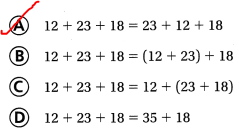Question 15. Use Symbols Complete the equation. 15 + (25 + 27) = (15 + ______) + 27 A. 40 B. 25 C. 10 D. 52 Answer: The given equation is: 15 + (25 + 27) = (15 + ______) + 27 Now, We know that, According to the Associative Property of Addition, If the groupings of addends changes, the sum stays the same So, 15 + (25 + 27) = (15 + 25) + 27 Hence, from the above, We can conclude that The symbol that will be used to complete the given equation is: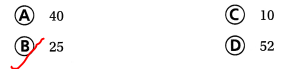Question 16. Multi-Step Nick read 24 pages in his book one day, 29 pages the next day, and 16 pages on the third day. Megan read 26 pages for three days in a row. Who read more pages? How many more? A. Megan read 9 more pages. B.Megan read 11 more pages. C. Nick read 9 more pages. D. Megan read 11 more pages. Answer: It is given that Nick read 24 pages in his book one day, 29 pages the next day, and 16 pages on the third day. Megan read 26 pages for three days in a row Now, The total number of pages read by Nick = (The number of pages read by Nick on the first day) + (The number of pages read by Nick on the second day) + (The number of pages read by Nick on the third day) = 24 + 29 + 16 = 40 + 29 = 69 pages Now, The total number of pages read by Megan = 26 + 26 + 26 = 78 pages Now, The difference between the number of pages read by Megan and Nick respectively = 78 – 69 = 9 pages Hence, from the above, We can conclude that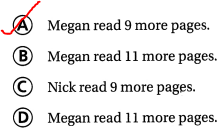Texas Test Prep Question 17. Canoes rent for$29 per day. Which expression can be used to find the cost in dollars of renting 6 canoes for a day?
A. (6 + 20) + (6 + 9)
B. (6 × 20) + (6 × 9)
C. (6 + 20) × (6 + 9)
D. (6 × 20) × (6 × 9)
It is given that
Canoes rent for $29 per day Now, The amount in dollars for renting 6 canoes for a day = (The number of canoes) × (The amount of rent in dollars for canoes per day) = 6 ×$29
= 6 × ($20 +$9)
= (6 × $20) + (6 ×$9)
Hence, from the above,
We can conclude that
The expression that can be used to find the cost in dollars of renting 6 canoes for a day is: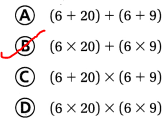### Texas Go Math Grade 5 Lesson 1.1 Homework and Practice Answer Key

Use properties to find the sum or product.

Question 1.
4 × 47
The given multiplication expression is: 4 × 47
Now,
4 × 47
= 4 × (40 + 7)                  Write 47 as a sum of a multiple of 10 and a number
= (4 × 40) + (4 × 7)          Use the Distributive Property
= 160 + 28                        Use Mental Math
= 188
Hence, from the above,
We can conclude that
The value for the given multiplication expression is: 188

Question 2.
3 × 40 × 6
The given multiplication expression is: 3 × 40 × 6
Now,
3 × 40 × 6
= (3 × 6) × 40                              Use the Associative Property of Multiplication
= 18 × 40
= 720
Hence, from the above,
We can conclude that
The value for the given multiplication expression is: 720

Question 3.
34 + 21 + 84 + 7
The given addition expression is: 34 + 21 + 84 + 7
Now,
34 + 21 + 84 + 7
= 34 + 7 + 21 + 84                           Commutative Property of Addition
= 41 + 105
= 146
Hence, from the above,
We can conclude that
The value for the given addition expression is: 146

Complete the equation, and tell which property you used.

Question 4.
103 + 21 = _______ + 103
The given equation is:
103 + 21 = _______ + 103
Now,
We know that,
According to the Commutative Property of Addition,
If the order of addends changes, the sum stays the same
So,
The completed equation is:
103 + 21 = 21 + 103
Hence, from the above,
We can conclude that
The completed equation is:
103 + 21 = 21 + 103
The property used to complete the given equation is: Commutative Property of Addition

Question 5.
16 + (70 + 8) = (16 + ______) + 8
The given equation is:
16 + (70 + 8) = (16 + ______) + 8
Now,
We know that,
According to the Associative Property of Addition,
If the grouping of addends changes, the sum stays the same
So,
The completed equation is:
16 + (70 + 8) = (16 + 70) + 8
Hence, from the above,
We can conclude that
The completed equation is:
16 + (70 + 8) = (16 + 70) + 8
The property used to complete the given equation is: Associative Property of Addition

Problem Solving

Question 6.
Maggie’s class bought three books at the book fair. The books cost $15,$9, and $12. Use parentheses to write two different expressions and find how much the class spent in all. Which property does the pair of expressions demonstrate? Answer: It is given that Maggie’s class bought three books at the book fair. The books cost$15, $9, and$12
Now,
The total amount of money the class spent in all = $15 +$9 + $12 = ($15 + $9) +$12                            Associative Property of Addition
= $15 + ($9 + $12) Associative Property of Addition =$15 + $21 =$36
Hence, from the above,
We can conclude that
The above pair of expressions demonstrate “Associative Property of Addition”
The total amount of money the class spent in all is: $36 Question 7. Leo’s basketball team makes 16 points in the first game, 22 points in the second game, and 18 points in the third game. Use the Associative Property of Addition to show two different ways Leo can group the addends to show the total number of points. Answer: It is given that Leo’s basketball team makes 16 points in the first game, 22 points in the second game, and 18 points in the third game Now, The total number of points earned = (The number of points the team makes in the first game) + (The number of points the team makes in the second game) + (The number of points the team makes in the third game) =$16 + $22 +$18
= ($16 +$22) + $18 Associative Property of Addition =$16 + ($22 +$18)                          Associative Property of Addition
= $16 +$40
= $56 Hence, from the above, We can conclude that The above pair of expressions demonstrate “Associative Property of Addition” The total number of points earned is:$56

Lesson Check

Question 8.
Which property is shown by the following equation?
44 + (13 + 11) = (44 + 13) + 11
D. Distributive Property
The given equation is:
44 + (13 + 11) = (44 + 13) + 11
Hence, from the above,
We can conclude that
The property that is showed by the given equation is: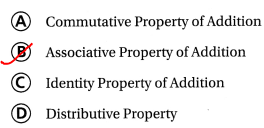Question 9.
Janel rakes leaves for $8 per lawn. Which expression can be used to find the amount she earns if she rakes 14 lawns? A. (8 + 10) × (8 + 4) B. (8 + 10) + (8 + 4) C. (8 × 10) × (8 × 4) D. (8 × 10) + (8 × 4) Answer: It is given that Janel rakes leaves for$8 per lawn
So,
The total amount of money she earned if she rakes 14 lawns = 14 × $8 =$8 × (10 + 4)
= (10 × $8) + (4 ×$8)
Hence, from the above,
We can conclude that
The expression that can be used to find the amount she earns if she rakes 14 lawns is: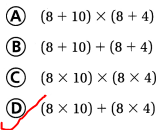Question 10
Sara bought 7 tickets to the school play. Each ticket costs $11. To find the total cost in dollars, she added the product 7 × 1 to the product 7 × 10, for a total of$77. Which property did Sara use?
A. Commutative Property of Multiplication
B. Associative Property of Multiplication
C. Identity Property of Multiplication
D. Distributive Property
It is given that
Sara bought 7 tickets to the school play. Each ticket costs $11. To find the total cost in dollars, she added the product 7 × 1 to the product 7 × 10, for a total of$77
Now,
The total cost of tickets in dollars = 7 × \$11
= 7 × (10 + 1)
= (7 × 1) + (7 × 10)
Hence, from the above,
We can conclude that
The property did Sara used is:Question 11.
There are 6 granola bars in each box. Which expression can be used to find the number of granola bars in a dozen boxes?
A. (6 + 10) × (6 + 2)
B. (6 × 10) + (6 × 2)
C. (6 + 10) + (6 + 2)
D. (6 × 10) × (6 × 2)
It is given that
There are 6 granola bars in each box
So,
The number of granola bars in a dozen boxes = 6 × 12
= 6 × (10 + 2)
= (6 × 10) + (6 × 2)
Hence, from the above,
We can conclude that
The expression that can be used to find the number of granola bars in a dozen boxes is: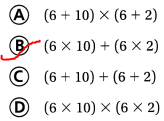Question 12.
Multi-Step Kara’s garden has 4 sections. Each section has 7 rows of carrots and 9 rows of celery. How many rows are in the garden?
A. 64
B. 56
C. 20
D. 36
It is given that
Kara’s garden has 4 sections. Each section has 7 rows of carrots and 9 rows of celery
Now,
The total number of rows in each section = 7 + 9
= 16 rows
So,
The total number of rows in 4 sections = 4 × 16
= 64 rows
Hence, from the above,
We can conclude that
The total number of rows that are present in the garden is: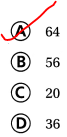Question 13.
Multi-Step Max’s school bought boxes of pencils. Each box holds 12 pencils. The fourth grade classes need 25 boxes. The fifth grade classes need 22 boxes. How many pencils are needed by both grades?
A. 300
B. 264
C. 564
D. 600
It is given that
Max’s school bought boxes of pencils. Each box holds 12 pencils. The fourth grade classes need 25 boxes. The fifth grade classes need 22 boxes
Now,
The total number of boxes = 25 + 22
= 47 boxes
So,
The number of pencils present in 47 boxes = 47 × 12
= (40 + 7) × (10 + 2)
= (40 × 10) + (40 × 2) + (7 × 10) + (7 × 2)
= 400 + 80 + 70 + 14
= 564 pencils
Hence, from the above,
We can conclude that
The number of pencils that are needed by both grades is: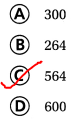Scroll to Top